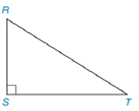Chapter 7.1, Problem 10EElementary Geometry For College St...

7th Edition
Alexander + 2 others
ISBN: 9781337614085

Solutions

Chapter
SectionElementary Geometry For College St...

7th Edition
Alexander + 2 others
ISBN: 9781337614085
Textbook Problem

Note: Exercises preceded by an asterisk are of a more challenging nature.In Exercises 3 to 8, use the drawing provided.Given: Right ∆ R S T Construct: The median R to S T -

To determine

To construct:

The median R to ST-

Explanation

Definition:

Perpendicular bisector of a triangle is a line passing through the midpoint of the particular side of the triangle.

Calculation:

The obtuse ABC is given

To construct a bisector we follow the below procedure.

Step 1: Construct a right angle triangle RST.

Step 2: Locate the midpoint of the side ST and label it as D.

Step 3: Draw a line from the point R to the point D

Still sussing out bartleby?

Check out a sample textbook solution.

See a sample solution

The Solution to Your Study Problems

Bartleby provides explanations to thousands of textbook problems written by our experts, many with advanced degrees!

Get Started

In Exercises 19-24, find the functions f + g, f g, fg, and fg. 19. f(x) = x2 + 5; g(x) = x2

Applied Calculus for the Managerial, Life, and Social Sciences: A Brief Approach

Determine the infinite limit. limx12x(x1)2

Single Variable Calculus: Early Transcendentals

True or False:

Study Guide for Stewart's Multivariable Calculus, 8th